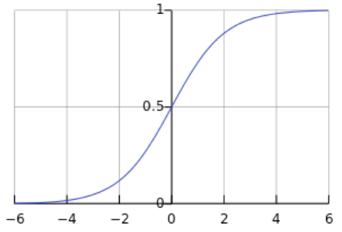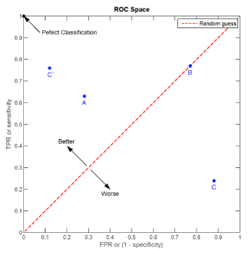Click in-app to access the full platform documentation for your version of DataRobot.

# Custom target expressions¶

Customizing target expressions provides one way to custom tune Eureqa models. Expressions may be any nested combination of Eureqa model building blocks. For example, if a, b, and c are input variables, example expressions might include:

• Target = 10 * a + b * c
• Target = if( a > 10, b, c) + 15

## What is the target expression?¶

The target expression tells DataRobot what type of model to create. By default, the target expression is an equation that models your target variable as a function of all input variables.

The target expression must be created in the form "Target = " regardless of the actual target variable name. For example, to create a target expression for target variable loan_is_bad (or default_rate, Sales, purchase_price, and so forth) you use the format "Target = f(...)". DataRobot automatically fills out the target expression for the selected Eureqa model type, using the defined target variable and input variables defined in the dataset. For a given target variable Target and 1 to n input variables of x, the default target expression for each of the search templates is:

• Numeric search template: Target = f(x1 ... xn)
• Classification search template: Target = logistic(f0() + f2() * f1(x1 ... xn))
• Exponential search template: Target = exp(f0(x1 ... xn)) + f1()

More complex expressions are also possible and give advanced users the power to specify and search for complex relationships, including the modeling of polynomial equations, and binary classification.

The Target Expression parameter, target_expression_string, is available within the Prediction Model Parameters and can be modified as part of tuning Eureqa models.

### Exponential search template¶

When DataRobot detects an exponential trend in the dataset for a Eureqa model, it applies the exp() function. As part of this process, DataRobot automatically takes the log() of all input variables, manipulates the transformed variables to get the final target value, and then uses exp() to invert the log transform.

The exp() building block is Disabled by default. If you are customizing the target expression in a model in a project whose data has an exponential trend, you may want to enable exp() for the model so that DataRobot will consider it during model building.

Tip

For Eureqa GAM models only: If you enable exp() support, you will want to select exponential as the variable for the EUREQA_target_expression_format parameter.

## Example expressions¶

The following are some examples of basic and advanced expressions you could create as target expressions. The examples below assume the dataset contains four variables named: w, x, y, and z.

### Basic examples¶

Model the Target variable as a function of variable x:

Target = f(x)

Model the Target variable as a function of two variables x and z:

Target = f(x, z)

Model the Target variable as a function of x and an expression, sin(z):

Target = f(x, sin(z))

Note

As shown in this example, including sin(z) and not z means DataRobot has access to the data in variable z only after it passes through the sine function.

### Multiple functions¶

To incorporate multiple functions into the target expression, use numbered functions starting with f0(). For example:

Target = f0(x) + f1(w, z)

Model the Target variable as a function of x, w, and the power law relationship:

Target = f(x, w, x^f1(), w^f2())

Find a mechanism change with two known models:

Target = if(x > f1(),exp(f2() * x), exp(f3() * x))

### Constrain the target expression format (GAM only)¶

For GAM models, you can enable the parameter EUREQA_target_expression_format if you want to constrain the expression format for the model. By default, there are no constraints to the expression format.

• exponential constrains the target expression to an exponential format, similar to the following:

Target = exp(f(...))

For example, if the default target expression would have been: Target = f(var1, var2, var3), the same target expression constrained to an exponential format would be: Target = exp(f(var1, var2, var3)).

• feature_interaction constrains the target expression to contain 2-way interactions detected between features as functions. This ensures the feature interaction is declared explicitly. For example, if the model detects interaction of features x and y, the expression will be:

Target = n + f(x, y)

(where n identifies other features of the dataset)

### Fit coefficients¶

You can represent an unknown constant or coefficient as a function with no arguments, f(). You can use multiple, no-argument functions, such as f1() to fit the coefficients of arbitrary nonlinear equations. For example, if you are looking for a polynomial of the form:

Target = a * x + b * x^2 + c * x^3

use the following target expression:

Target = f0() * x + f1() * x^2 + f2() * x^3

### Nested functions¶

Model the Target variable as the output of a recursive or iterated function to a depth of 3:

Target = f(f(f(x)))

## Binary classification¶

If y is a binary variable filled with 0s and 1s, model it using a squashing function, such as the logistic function. Using DataRobot for classification has a few advantages:

• Finding models requires less data
• Models can often extrapolate extremely well
• Resulting models are simple to analyze, refit, and reuse
• The structure of the models gives insight into the classification problem, allowing you to both predict as well as learn something about how the classification works

### Basic binary classification¶

Model the Target variable as a binary function of x and w:

Target = logistic(f(x, w))

Keep in mind that the logistic function will produce intermediate values between 0 and 1, such as 0.77 and 0.0001; therefore, you will need to threshold the value to get final 0 or 1 outputs.## Model constraints¶

You can also use the target expression to constrain the model output. You can include require and/or contains functions in target expressions to force specific model building or output behaviors.

Tip

Be aware that require and contains are very advanced, "experimental" settings that make it harder for DataRobot to find solutions and may significantly slow model search. To use these settings, we strongly suggest that you contact DataRobot for assistance as output behavior cannot be guaranteed.

If you need to force a certain variable or term to appear in Eureqa models, add a term that nests require or contains functions.

Model y as a function of x, with all projects required to contain an x^2 term:

Target = f(x) + 0 * require(contains(f(x),x^2))

For this to work, the first term of the contains operator must exactly match the functional term you are trying to fit (f(x) in this case). By multiplying the second term by 0, you guarantee that it won't impact the value produced by a particular solution f(x).

You can also enforce a constraint on the model output if there are certain realities that need to be followed (e.g., price > cost). To do this, add a term with the require function.

Model y as a function of x, with all solutions required to output values greater than 0:

Target = f(x) + require( f(x) > 0 )

The following is a faster alternative:

Target = max( f(x), 0 )

### Force a condition¶

There may be known relationships in your data that do not fully explain the data. For example, if you have a model that was generated based on academic theory, you can use DataRobot to fit the residual between that known model and the actual data.

Model Target as a function of x, using existing knowledge of an x^2 relationship to model the residual:

Target = f0(a, b, c, d, e) + f1() * x^2

DataRobot will interpret f1() as a coefficient and fit the term appropriately.

Updated October 26, 2021